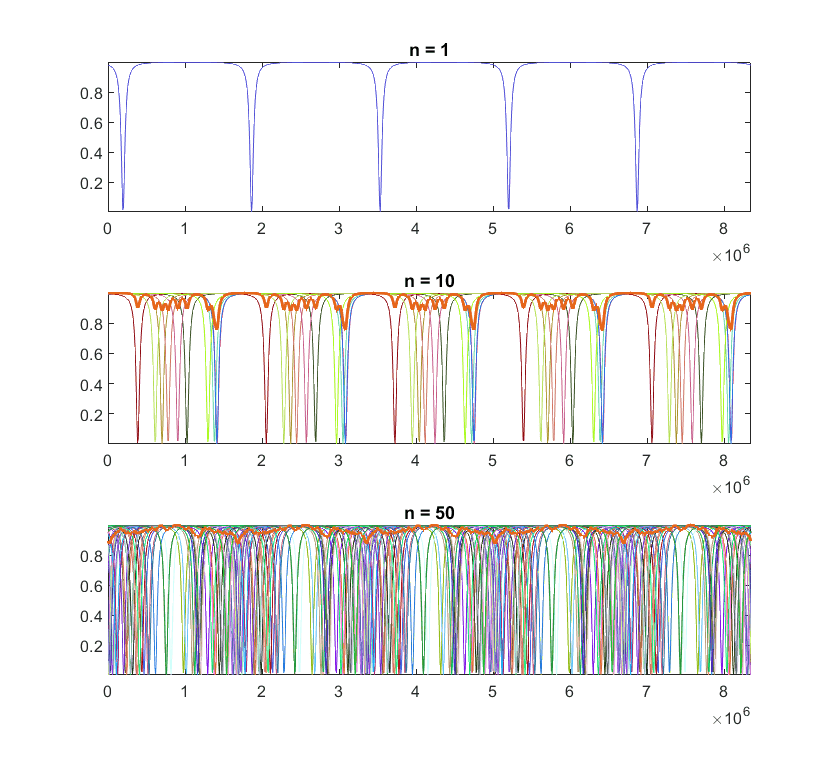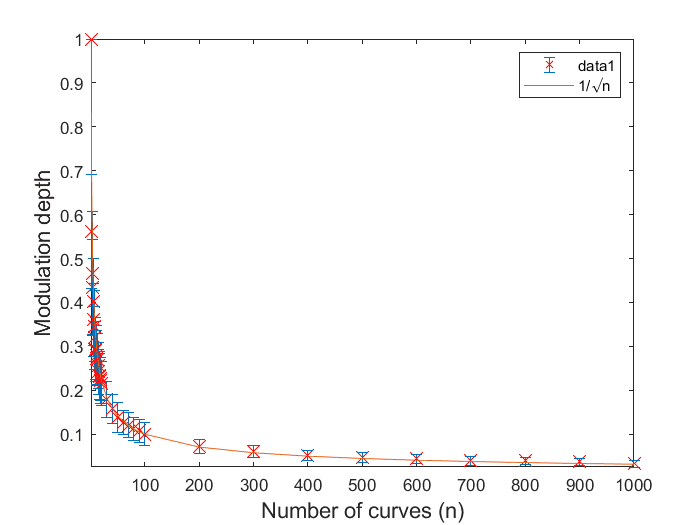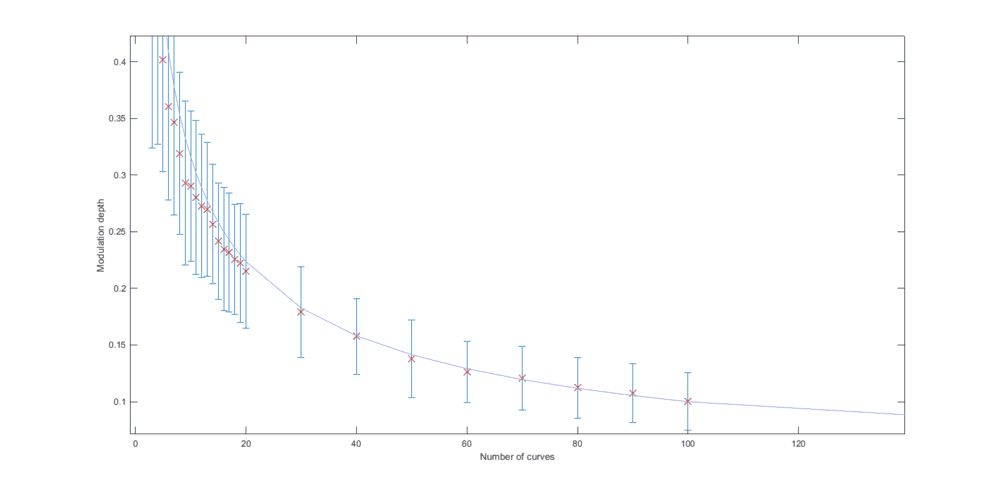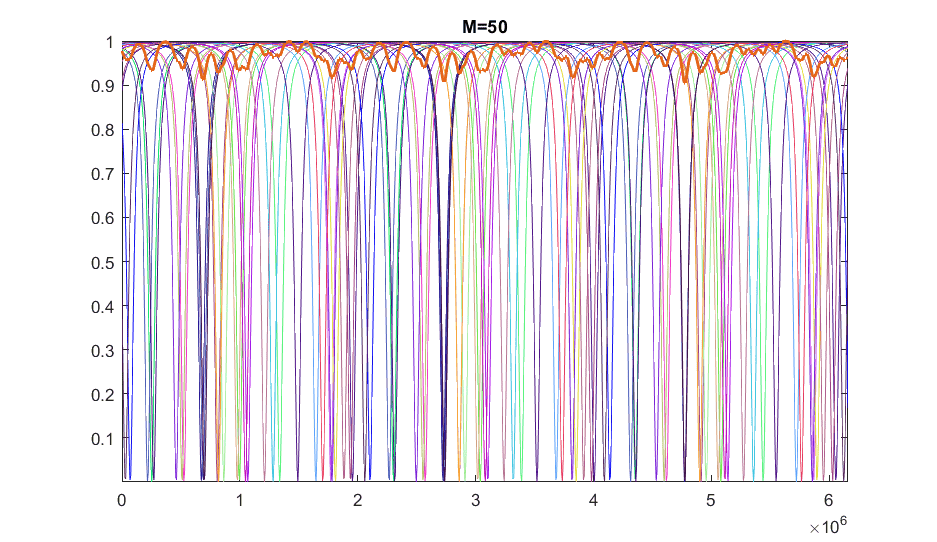# Central Limit Theorem and fitting data

• I
• roam
So the "clt" for a family of functions of the max and min functions is not obvious.In summary, the conversation discusses a simulation that generates multiple identical curves with different phases and calculates their sum. It is observed that as the number of curves increases, the fluctuation depth decreases as expected. The simulation also shows that the fluctuation depth is proportional to the standard deviation, which is in line with the Central Limit Theorem. However, the discussion raises questions about the applicability of CLT in this situation, particularly due to the dependence of the individual curve components and the complexity of the modulation depth function.

#### roam

TL;DR Summary
Why does the curve ##1/\sqrt{n}## best fit my simulation data shown below? How does my simulation relate to the Central Limit Theorem?
I am working with a simulation which generates an arbitrary number ##n## of identical curves with different phases and calculates their (normalized) sum. As expected, the fluctuation depth of the curves decreases as we increase ##n##. Here is an example of my simulation (when ##n>1##, the thicker line is the final sum):When I plot the fluctuation depth versus ##n##, I find that they vary according to:

$$\frac{1}{\sqrt{n}} \tag{1}$$

The following are my measurements fitted with ##1/\sqrt{n}##. Each data point is the average of 100 runs and the error bars show the standard deviation.So, how do we make the connection between my simulation results and the Central Limit Theorem?

It appears that the fluctuation depth is proportional to its standard deviation ##\sigma##. And from the Central Limit Theorem, we know that the standard deviation varies according to ##1/\sqrt{n}##, where ##n## is the sample size.

Any explanations would be greatly appreciated.

The central limit theorem applies when the samples are independent, which they are in your simulation. Your results seem to be as expected.

•roam
Hi mathman,

By samples do you mean the individual curves or the standard deviations associated with each average?

The individual curves are the samples.

•roam
According to CLT, sample SD will approach ## \sigma/\sqrt n ## , and, after normalizing, you have ##\sigma =1 ##.

•roam
roam said:
Summary: Why does the curve ##1/\sqrt{n}## best fit my simulation data shown below? How does my simulation relate to the Central Limit Theorem?
People have supplied intuitive answers. However, if you want a rigorous mathematical analysis, this could be complicated.

I am working with a simulation which generates an arbitrary number ##n## of identical curves
This doesn't define a specific probability model because "curve" isn't specifically defined. If you generated a "curve" f(x) by taking an independent random sample at each location x on the curve, most of your "curves" wouldn't have smooth sections in them.

As expected, the fluctuation depth of the curves decreases as we increase ##n##.

What is the definition of "fluctuation depth"?

The Central Limit theorem applies to the sum of independent identically distributed random variables. Your problem involves random vectors whose components appear to be dependent and it is apparently not a question about a sum of numbers, but rather about a difference between the max and min of a set of numbers.

•roam
Hi @Stephen Tashi

Thanks a lot for your post.

Stephen Tashi said:
What is the definition of "fluctuation depth"?

The Central Limit theorem applies to the sum of independent identically distributed random variables. Your problem involves random vectors whose components appear to be dependent and it is apparently not a question about a sum of numbers, but rather about a difference between the max and min of a set of numbers.

Yes, I have defined the "fluctuation depth" (or "modulation depth") of a curve ##m## as:

$$\text{modulation depth}=\frac{m_{\text{max}}-m_{\text{min}}}{m_{\text{max}}}.$$

What do you mean by the components of the vectors being dependent? The indivudual vectors are generated by the "Airy function" which is basically a sum of Lorentzians.

But the individual underlying vectors have slightly randomly different phases and periods (so the resulting combined curve will have a period equal to the least common multiple of the individual periods). For a given number of curves ##n##, each time I run the simulation, the curves will overlap differently to produce a somewhat different fluctuation depth. But when I run the simulation many times for different ##n##, modulation depth clearly varies according to the Central Limit Theorem. So, why do you think Central Limit theorem applies so well to my situation?

The CLT applies to the average of random samples from a distribution. Are you averaging something (either directly or by as a result of your process)? If you are, then your results probably are due to the CLT. Otherwise, probably not. I don't see any averaging, but I'm not sure that I really understand your process.

CORRECTION: I forgot that the thick-line curve is the normalized sum (average), not the maximum of the individual curves. Therefore, each point on that curve is the average result of a sample. The CLT applies to each point. Because of the randomness of the phase shift, I believe that the curve points in their entirety represent a large sample of such experiments and should approach the normal distribution due to the CLT.

CORRECTION TO CORRECTION: the individual points of the normalized sum curve are not independent. So they can not necessarily be considered an independent sample that should satisfy the normal distribution.

Last edited:
•roam
roam said:
What do you mean by the components of the vectors being dependent?

One vector is one curve. It is defined by a function ##f(x)##. For each ##x##, regard ##f(x)## as one component of the vector ##f##. The simplest way to see that the components are dependent is to ask what graphs of the functions would look like if you chose the value of each ##f(x_1)## independently of each ##f(x_2)##. If you did things in such a manner, you would choose ##f(x_1)## from some probability distribution and choose ##f(x_2)## independently from some, perhaps different, probability distribution. If you try generating curves that way, you will see they don't resemble the curves in your example.

My guess about what you are doing is that you have some family of functions ##f(x,a,b,c,...)## that are defined by parameters ##a,b,c,...## and you are picking "random" functions by picking ##a,b,c,..## from some probability distributions. This method of generating functions, does not make ##f(x_1, a_0, b_0, c_0,...)## independent of ##f(x_2, a_0, b_0, c_0)## for a given choice of ##a_0, b_0, c_0##.

As to the definition of "modulation depth", if you are familiar with the concept of "order statistics", you know that the distribution of the max and min functions associated with a N samples from a distribution are not simple algebraic functions of the sum of the values of the samples. The distribution of a function of the max and min functions could be even more complicated.

•roam
Hi @Stephen Tashi and @FactChecker

Thanks a lot for the explanation. I can see why the individual points on the sum are not independent.

Here is a close up of my data fitted with ##\frac{1}{\sqrt{n}}##. As one can see, the ##\frac{1}{\sqrt{n}}## estimate becomes more accurate as ##n## (the number of underlying curves/samples) is increased:Is it completely coincidental that the data seem to follow the normal distribution? Or does CLT still apply to some extent?

Stephen Tashi said:
My guess about what you are doing is that you have some family of functions ##f(x,a,b,c,...)## that are defined by parameters ##a,b,c,...## and you are picking "random" functions by picking ##a,b,c,..## from some probability distributions. This method of generating functions, does not make ##f(x_1, a_0, b_0, c_0)## independent of ##f(x_2, a_0, b_0, c_0)## for a given choice of ##a_0, b_0, c_0##.

Yes, that means that the minimum and maximum points on any given curve are not independent.

I think we are more interested in the two points ##f(x_1, a_0, b_0, c_0)## and ##f(x_1, a_1, b_1, c_1)##. As @mathman said, these are the individual samples that need to be independent. I think these are still dependent but to a lesser extent.

Stephen Tashi said:
As to the definition of "modulation depth", if you are familiar with the concept of "order statistics", you know that the distribution of the max and min functions associated with a N samples from a distribution are not simple algebraic functions of the sum of the values of the samples. The distribution of a function of the max and min functions could be even more complicated.

I have very limited experience with order statistics. I am simulating a real world situation where the measured signal is the superposition of many individual signals. But we don't know much about the individual curves, the final sum is the only thing that we can measure. Do you know of a better analytic expression for modulation depth?

roam said:
Here is a close up of my data fitted with ##\frac{1}{\sqrt{n}}##. As one can see, the ##\frac{1}{\sqrt{n}}## estimate becomes more accurate as ##n## (the number of underlying curves/samples) is increased:

View attachment 242975

Is it completely coincidental that the data seem to follow the normal distribution? Or does CLT still apply to some extent?

Let's be specific about what the data is.

I think a red "x" is computed as follows. We fix the number of curves N to be added to produce 1 sample curve. We generate M such sample curves independently. We compute the modulation depth for each sample curve. We take the mean of those modulation depths. This mean value gives us the y-coordinate of the red "x".

The red "x" is the value of the "sample mean" of M independent samples taken from the same distribution The CLT says that the standard deviation of this sample mean (considered as a random variable) is proportional to ##\frac{1}{\sqrt{M}}##. It doesn't say anything (directly) about how the sample mean depends on ##N##.

So the CLT says something about the size of the "error bar" around the red "x". It doesn't say anything about the y-coordinate of the red "x".

One can think of ways to generate "random" curves that would not produce the results in your data. For example, suppose we generate a random curve ##F## on ##[0,1]## by adding ##N## curves, each of which is given by a function whose graph is a straight line from ##(0,0)## to ##(u,1)## and another straight line from ##(u,1)## to ##(1,0)## where ##u## is a random variable uniformly distributed on [0,1].

Such a curve ##F## has a max value of ##N## and a min value of 0. So its modulation depth is ##(N-0)/N##, which is 1, regardless of how big we make ##N##.

It wouldn't surprise me if the CLT can play a role in analyzing your results, but it isn't straightforward to see how. Your results depend on generating the random curves is a specific way.

•roam
Hi @Stephen Tashi

Thanks for the explanation. Yes, this is exactly how the means (the red "x"s) are calculated.

But I am a little confused, aren't M and N the same thing here? In my plot, the values on the abscissa are the number of individual curves (i.e., the samples size) that were added to produce 1 final curve.

roam said:
But I am a little confused, aren't M and N the same thing here? In my plot, the values on the abscissa are the number of individual curves (i.e., the samples size) that were added to produce 1 final curve.

In my notation ##M## and ##N## are different. Suppose ##N = 50##. The red "x" has coordinates of about (50, 0.15). Where does the "error bar" around the point (50, 0.15) come from? What determines it's length? I'm assuming you generated some large number ##M## of samples, each sample of which was a curve formed by adding 50 random curves. So ##M## didn't have to be 50.

Are you saying that you set ##M = 1##?

•roam
Stephen Tashi said:
In my notation ##M## and ##N## are different. Suppose ##N = 50##. The red "x" has coordinates of about (50, 0.15). Where does the "error bar" around the point (50, 0.15) come from? What determines it's length? I'm assuming you generated some large number ##M## of samples, each sample of which was a curve formed by adding 50 random curves. So ##M## didn't have to be 50.

Are you saying that you set ##M = 1##?

Thanks. The distinction is clear now. In my experiment, I set ##M## at a fixed value of ##100##.

So, according to CLT we expect the size of the error bars to be proportional to ##\frac{1}{\sqrt{M}}##. But if you look at the plot in my first post, the error bars get smaller as ##N## gets larger. So it appears to me that the standard deviation is clearly related to ##N##. Is there an explanation for this?

roam said:
So, according to CLT we expect the size of the error bars to be proportional to ##\frac{1}{\sqrt{M}}##. But if you look at the plot in my first post, the error bars get smaller as ##N## gets larger.
The CLT only says that if you are taking ##M## samples from the same random variables then the standard deviation of the sample mean is proportional to ##\frac{1}{\sqrt{M}}##. It doesn't tell us how to compare the standard deviations of the sample means of M=100 samples taken from two different random variables. When you change ##N##, you change the random variable that you are considering.

So it appears to me that the standard deviation is clearly related to ##N##. Is there an explanation for this?

I think there is an explanation, but the explanation is not given by a simple and straightforward use of the CLT. The explanation must incorporate the particular way that you picking the "random" curves to add. Communication engineers have probably studied the statistics of a signals resulting from adding waves of random phases. Perhaps someone like @Baluncore can tell us their approach to analyzing the problem.

•roam
Stephen Tashi said:
Communication engineers have probably studied the statistics of a signals resulting from adding waves of random phases. Perhaps someone like @Baluncore can tell us their approach to analyzing the problem.
“The only interesting answers are those which destroy the question”. —Susan Sontag
Look at the psychology, not the math. I would assume there was a reason for simulation of the situation, and that the fascination with the CLT is a red herring. You need to throw the windows open, look at the horizon, get your bearings, then dive in at the deep end.

When the current approach does not yield results, go far back to the first principles. I would look at the engineering or algorithm that generates the n=1 signal in post #1. I would question the shape of that fundamental curve and identify the harmonic components. I would want to know what decides the phase, or triggers the timing.

•roam
Hi @Baluncore

The formula that generates a single curve is:

$$\frac{a^{2}-2ba\cos\varphi+b^{2}}{1+b^{2}a^{2}-2ra\cos\varphi},$$

where the constants ##a,\ b## are fixed throughout the course of the experiment. The only thing that varies is the phase term ##\varphi## given by another equation:

$$\varphi=n c \omega,$$

where ##c## is another fixed constant, ##\omega## are the x-axis points (from a vector), and ##n## is a random number. Numerically, ##n## is made to be in the interval 0.001 away from 1.5 in the positive side.

The algorithm generates an arbitrary number ##N## of these curves by generating ##N## random values of ##n## and substituting them into the equation above. Therefore, each curve is generated by a different ##\varphi## vector, so they each appears to have a slightly different phase/frequency. When ##N>1##, the final curve is calculated by adding all the individual curves point by point.

Do you believe it is because of the way the algorithm was written that the modulation depth varies according to ##1/\sqrt{N}##?

P.S. To avoid confusion with ##n## in my formula (representing group index of light), I am using ##N## for the number of individual curves to be added.

I assume the -2ra Cos() should read -2ba Cos() in the equation.

The signal is critically dependent on the values of a and b.
What values of a and b were used for the first signal in post #1.

The value of c sets the x scale and amplifies the random phase.
What value do you have for c in that first post.

Regarding the random ( 1.5 < n < 1.501 ).
How is that value distributed during your simulation, is it linear?

•roam
Hi @Baluncore

Yes, that "r" is meant to be a "b". Sorry, that was a typo.

I used the fixed values ##a = b = 0.95##.

##c=3.3 \times 10^{-7}## is also a physical constant that does not change.

I believe the random ##n## is a uniformly distributed random number. In MATLAB this is generated using:

Code:
n = 1.5 + 0.001*rand(1,1)

When I change the parameters ##a, b##, the sharpness of the dips change (in terms of the physics ##a=b##, ##a<b##, and ##a>b## each represent different regimes). However, the modulation depth still obeys the ##1/\sqrt{N}##. Why?

OK, your signal is the same function every time, but the phase is different.
There is no additive phase term in your φ=ncω, so everything is in phase at time ω = zero.
Should that phase not be something more like φ=n+cω.

Is Rnd(1,1) a self defined random number?
If so how is it defined, or why are there two parameters?

Last edited:
Yes, that is correct.

No, I did not include an additive phase term.

My simulation relates to optical resonators, where ##\varphi## represents the phase difference imparted by a round trip propagation through the cavity. So, we can assume they all start off in phase, but acquire different phases later on as they propagate.

The actual physical equation that relates phase shift to frequency and ##n## is:

$$\varphi=n \ \omega \frac{ \ \text{Length}}{\text{speed of light in vacuum}} = n \ \omega \ c.$$

roam said:
So, we can assume they all start off in phase, but acquire different phases later on as they propagate.
From post #1, plots for n=10 and n=50, we see that the phase shift is not distributed linearly over 360°. The maximum of the normalised accumulated signals will be biased closer to 1 than expected because there are some values of w that see very few nearby dips. The minimum will be where more dips are concentrated. Without knowing the actual range and distribution of the phase variation it is not possible to push the analysis any further.

You cover approximately 5 cycles, so you are repeating the simulation arithmetic. It might be simpler and quicker to cover only one cycle.

Since you are summing signals with independent random phase I would expect the CLT to be applicable.

•roam

Do you have an idea on how CLT applies to this situation?

##M## is the number of curves added to generate one curve (i.e., the sample size). So, according to CLT, the standard deviation of this sample's mean varies proportionally to ##1/\sqrt{M}##. As expected, we can see from the plot in my first post, the error bars do get smaller as ##M## is increased.

But the problem is that CLT should only be able to predict the size of the error bars, not the location of the y-coordinates (means). In my plot, the ordinate represents the mean modulation depth. My results show that this mean is readily calculable from CLT (##\text{mean modulation depth} \approx 1/\sqrt{M}##). How is that possible?

Baluncore said:
From post #1, plots for n=10 and n=50, we see that the phase shift is not distributed linearly over 360°. The maximum of the normalised accumulated signals will be biased closer to 1 than expected because there are some values of w that see very few nearby dips.

I think that might have been a coincidence. When I run the code many times, often the phase shift is nearly linearly distributed. For instance: